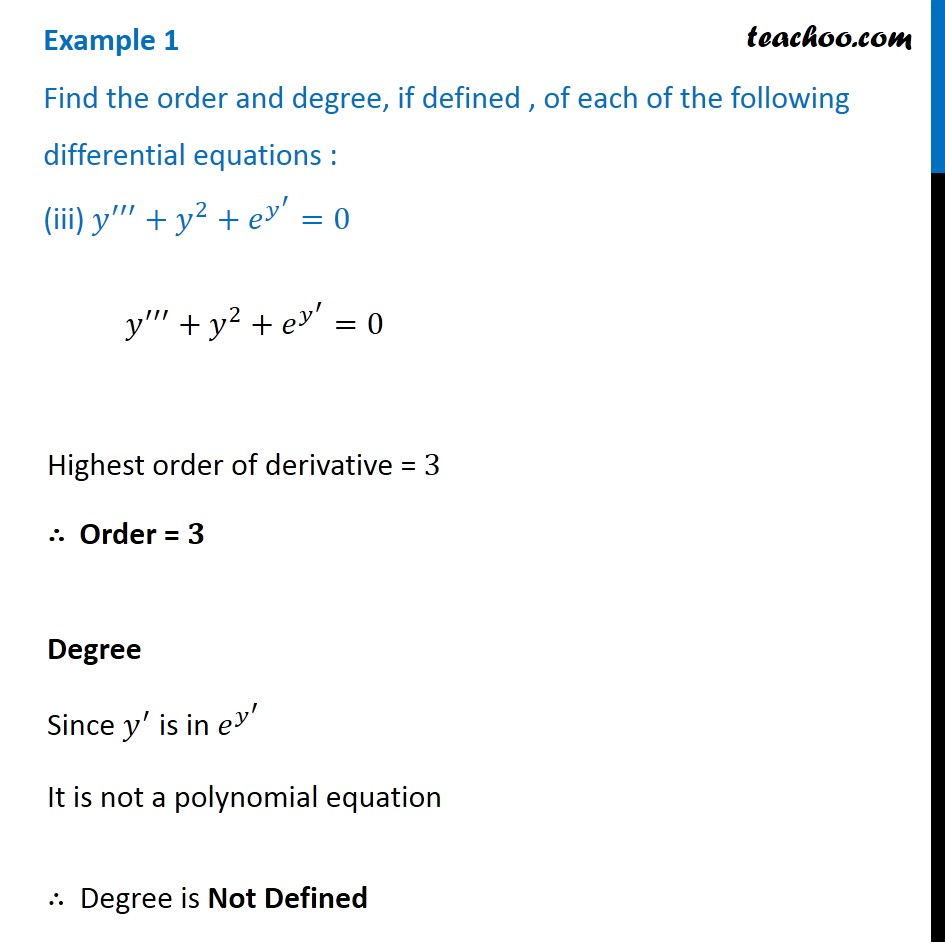Examples

Chapter 9 Class 12 Differential Equations
Serial order wiseMaths Crash Course - Live lectures + all videos + Real time Doubt solving!

### Transcript

Example 1 Find the order and degree, if defined , of each of the following differential equations : (iii) 𝑦^′′′+𝑦^2+𝑒^(𝑦^′ )=0 𝑦^′′′+𝑦^2+𝑒^(𝑦^′ )=0 Highest order of derivative = 3 ∴ Order = 𝟑 Degree Since 𝑦^′ is in 𝑒^(𝑦^′ ) It is not a polynomial equation ∴ Degree is Not Defined